News

# MOV Selection Guide | Power Supply Peripheral Design Series

In the power supply application, to ensure the stability and the safety of the power supply, peripheral protection circuits are often designed to ensure that the power supply is not damaged during use. Peripheral circuits include surge protection circuit, electrostatic protection circuit, EMI filter circuit, and pulse group anti-interference circuit. This paper will discuss the use and selection methods of MOV applied in surge protection circuits.

Ⅰ. What are Metal Oxide Varistors?

MOV stands for Metal Oxide Varistors, a voltage-limiting protection device with nonlinear volt-ampere characteristics. It is a matrix structure composed of zinc oxide particles. The grain boundaries between the particles have electrical characteristics similar to bidirectional PN junctions.

Application of MOV: MOV is often used in parallel with the protection device to clamp the voltage when the circuit is subjected to overvoltage, absorbing excessive current to protect the subsequent circuit.

Main characteristics of MOV: when the voltage applied at both ends is within the nominal rating, its resistance is almost infinite and in a high resistance state, and the leakage current is as low as tens of microamperes. When the voltage applied at both ends slightly exceeds the nominal rating, its resistance drops sharply, and it is immediately turned on, with working current increasing by several orders of magnitude, and the response time as low as nanoseconds. When the voltage applied at both ends exceeds its maximum limit voltage, MOV will be completely broken down and cannot recover by itself.

In normal use, the varistor, in the leakage current zone, enters the nonlinear zone to discharge the surge current, when impacted by the surge, and generally cannot enter the saturation zone. When the varistor is in low voltage, it works in the leakage current area, showing a large resistance, and the leakage current is very small; When the voltage rises to the non-linear region, the voltage changes little. Then the current changes in a considerable range and the voltage limit is better; When the voltage rises again, the varistor enters the saturation zone, showing a small linear resistance, and the current is large. Over time, the varistor will overheat and burn or even burst. Therefore, the varistor cannot withstand a current of 1mA for a long time.

Characteristics of MOV：

(1) Wide working voltage range (mainstream 18-1800V, divided into several grades). According to the market mainstream, it is mainly as follows:

l  5D——V1mA：18~750V

l  7D——V1mA：18~820V

l  10D——V1mA：18~1100V

l  14D——V1mA：18~1800V

l  20D——V1mA：18~1800V

l  25D——V1mA：18~1800V

(2) Fast response to overvoltage pulse (single-digit to tens of nS);

(3)  Strong impulse current resistance (up to 100-15000A). According to the market mainstream, it is mainly as follows:

l  When 5D——V1mA=18~56V，I=100A；When V1mA=68~750V，I=400A

l  When 7D——V1mA=18~68V，I=500A； When V1mA=82~820V，I=1750A

l  When 10D——V1mA=18~68V，I=500A；When V1mA=82~1100V，I=2500A

l  When 14D——V1mA=18~68V，I=1000A；When V1mA=82~1800V，I=4500A

l  When 20D——V1mA=18~68V，I=2000A；When V1mA=82~1800V，I=6500A

l  When 25D——V1mA=18~68V，I=4500A；When V1mA=82~1800V，I=15000A

(4) Small leakage current (lower than single-digit to dozens of uA);

(5) Low resistance temperature coefficient.

Ⅱ. Naming Rules of MOV

The MOV is named as shown below: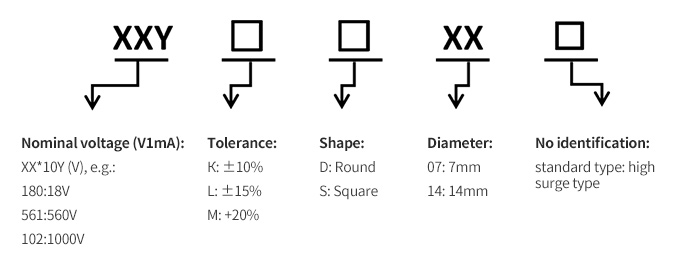No identification: standard type: high surge type

For example, 471KD14 means the nominal voltage is 470VDC; the tolerance is ±10% (which means the operating voltage is between 423~517VDC); the shape is round, and the diameter is 14mm.

Ⅲ. Specification Calculation

(1) Step 1: VARISTOR VOLTAGE calculation

V1mA=1.5Vp=2.2Vnom                （1）

In this formula, Vp is the peak value of the circuit's rated voltage, and Vnom is the effective value of the rated AC voltage.

For example: when a client uses MORNSUN LHE60-20B12 power supply products, the rated input voltage is 220VAC, then the varistor voltage value V1mA=1.5Vp=1.5×1.414×220V=476VDC, V1mA=2.2Vnom=2.2×220V=484VDC, so The breakdown voltage V1mA of the varistor can be selected between 470V and 480V, such as 470V for V1mA, corresponding to 471KDxx varistor.

(2) Step 2: Calculation of MAXIMUM ALLOWABLE VOLTAGE

This voltage is divided into two types: AC (VACrms) and DC (VDCr):

VACrms≈0.64V1mA                （2）

VDCr≈0.83V1mA                   （3）

Taking the example of step 1, and the calculation steps are as follows:

V1mA=470V, then VACrms≈0.64V1mA=300VAC, VDCr≈0.83V1mA=390VDC. VACrms that the 471KDxx varistor corresponds to is 300V, VDCr=385V, the verification of the parameters calculated in step one is feasible.

(3) Step 3: Calculation of flow capacity (I (8/20us))

The maximum flow rate is proportional to the diameter of the varistor. When selecting the diameter of the varistor, the lightning surge level of the protected circuit is first determined, on which basis, the maximum flow rate of the varistor is selected.

When the varistor impact test is performed, as the number of impacts increases, the impact current applied each time should be reduced accordingly. Generally, the current technical specifications present value I for one varistor shock. Therefore, in general, when we select a varistor, the flow capacity should be calculated by pressing the 10 to 100 impacts in the rating curve of the varistor surge lifetime. The actual surge current passed is about 30% of the maximum impact current. (Ie 0.3I).

If the flow rate required by the surge level is not known, it can be calculated with the differential mode voltage across the varistor according to the figure below.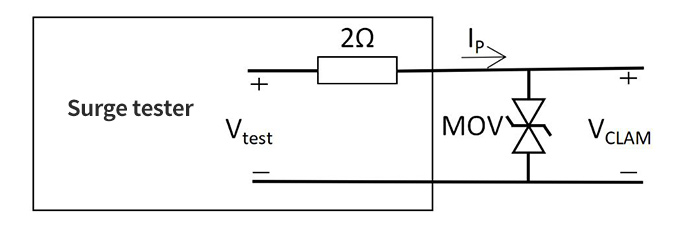For example surge differential mode voltage Vtest=2KV, varistor 471KDxx maximum limit voltage VCLAM=775V, then the flow calculation is as follows:

I P= (Vtest - VCLAM) / 2 = 612.5A        （4）

I=IP/0.3=2042A                             （5）

The flow rate corresponding to the 471KD10 varistor (referring to the Withstanding Surge Current column of the varistor specification) is 2500A, which is greater than 2042A; the flow capacity I corresponding to the 471KD07 varistor is only 1750A; therefore, in the case of step 1, the varistor is recommended to be more than 10D, such as 471KD10.

Ⅳ. Key Indicators

1) Nominal varistor voltage (V1mA) refers to the voltage value across the varistor when passing a pulse current of a specified duration (generally the duration of 1mA is generally less than 400mS);

2) Maximum limit voltage (VCLAM) refers to the residual voltage which means the maximum pulse peak current Ip that the varistor can withstand and the peak voltage across the varistor under the specified waveform;

3) Flow capacity (IP) refers to the maximum pulse (peak) current value allowed to pass through the varistor under specified conditions (such as in specified time interval and number of times, and applying standard impulse current);

4) Leakage current refers to the current value flowing in the varistor when the maximum continuous DC voltage is applied under the condition of 25℃;

5) Grade voltage refers to the voltage peak value that appears at both ends of the varistor when it passes 8/20 grade current pulses.

Ⅴ. Notes

1) Response time: The response time of the varistor is ns, which is faster than gas discharge tubes and slightly slower than TVS tubes;

2) Junction capacitance: The junction capacitance of the varistor is generally in the order of hundreds to thousands of pF. In many cases, it is not suitable to be directly applied to the protection of high-frequency signal lines. Because its large junction capacitance will increase the leakage current, when it is applied in the protection of AC circuits, the design of the protection circuit needs to be fully considered;

3) Flow capacity: The flow capacity of the varistor is larger but smaller than the gas discharge tube;

4) Circuit position: Varistor is generally used between the L line and N line of the input end of the product, which is between the fuse and thermistor;

5) Residual voltage: The residual voltage of the varistor (ie the maximum limit voltage) will be directly applied to the back-end circuit of the product. Excessive residual voltage may cause damage to back-end devices (such as rectifier bridges or large capacitors), so the design must ensure that the residual voltage does not exceed the maximum voltage that the subsequent circuit can withstand;

6) When designing the circuit, the projection of the input varistor should be far away from the control IC to prevent the IC from being interfered with during the surge test, causing the output voltage to lose power during the surge test.

VI. Measurement of Varistor

Separate measurement: Usually varistor has good nonlinearity, which means the resistance decreases with the increase of the applied voltage, and it features a bidirectional current. When measuring, place the multimeter in the R×1k block, and measure the positive and negative insulation resistance between its two pins. Both should be infinite, otherwise, the leakage current is too large. Small measured resistance represents that the varistor is damaged and cannot be used. The varistor can be measured with a digital multimeter.

In the use of power supply, peripheral circuits are indispensable, and it is particularly important to select a suitable MOV for surge protection circuits. What other key points of the peripheral circuit need to be paid attention to? Welcome to follow MORNSUN’s public account, we will keep update!

PromptSuccessful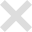Contact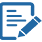MORNSUN distributors for part ""
Distributor Stock Qty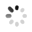NAC Group, Inc. 0 Buy online

Find the right contact in your region